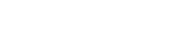📜

Notes:

• //What we can currently do is to convert a small part of the mass of uranium. This is why Einstein's equation was instrumental in developing nuclear fission technology, used for nuclear weapons and nuclear power. By measuring the mass of the nuclei of an atom (that is heavier than [iron (https://ideas.discover.earth/iron/)), and subtracting the mass of its component protons and neutrons, you can calculate the 'binding energy' of the nucleus, or the energy that it takes to keep the large atom stuck together. It is part of this binding energy that is released as explosive heat and radiation when the atom is split.//
• //This is exactly what Albert Einstein's famous E=mc2 equation calculates: how much energy (E) an object with mass has. The answer is the mass of the object (m) times the speed of light (c) squared and considering the enormous value of the speed of light; it’s quite a lot.//
• //For reference, the atomic bomb dropped on Nagasaki released 84,000,000 MJ. If we managed to detonate an average sized 62 kg person and set all of that energy free, they'd explode with the force of 66,000 nuclear bombs and probably destroy the planet, or power civilisation for years.//
• Matter is energy, and can be converted to other forms.
• Theoretically, every atom in your body could be converted into an explosion of light and gamma radiation.
• It seems that energy has a number of manifestations. One of them is matter (which has mass). Another is electromagnetic radiation.
• The hunt for the Higgs-Boson at the LHC and similar experiments is trying to determine the relationship between energy and mass. I think that there's some kind of field which interacts with particles that have certain properties, which causes mass.
• Once they work this out, perhaps we can create hydrogen from energy. The other step is creating other atoms from hydrogen, which is called fusion.
• Have a read of this article below. We're still waiting for the results they claim many years later, but I do think that even the fact that it is theoretically possible is astounding.
• Matter will be created from light within a year, claim scientists
• Mass is a form of energy.
• Just like other forms of energy, the mass of an object itself can be converted into another form of energy. Although there are no known theoretical methods of doing that that are practical, one such method is to annihilate matter with antimatter.
• Related are the fusion and fission of atoms. Fusing elements below iron (fusion) and splitting atoms above iron (fission) converts some of the mass of the original particles into radiant energy. The latter is the basis of nuclear power.
• E=mc2 is how much energy that an object with mass has. It's the mass times the speed of light squared, and considering how huge the speed of light is, it’s quite a bit.
• An interesting implication of this is that if you get an object at rest and heat it, you can measure its mass increase (with a sensitive enough scale)
• The mass–energy relation E = mc2 can be used to relate the rest energy to the rest mass, or to relate the total energy to the total mass. To instead relate the total energy or mass to the rest energy or mass, a generalization of the mass–energy relation is required: the energy–momentum relation.
• In other words when momentum gets involved you have to use this equation:• Intrinsic rest mass m0, total energy E, and a momentum of magnitude p, where the constant c is the speed of light.
• Electromagnetic radiation is another expression of energy. It has no mass, and travels at the speed of light. It’s momentum of magnitude (p) is massive. Thus E is pretty big.
• [I don’t think this necessarily means that mass and electromagnetic radiation are on the same spectrum and can be readily converted into one another, just that they are both expressions of energy. But cannot one be converted into energy, and then into the other? What exactly is energy?]